# Python, Pillow: Rotate image

Posted: 2019-06-25 / Modified: 2021-04-06 / Tags: Python, Pillow, Image Processing

In the `Image` module of the Python image processing library Pillow(PIL), a method `rotate()` for rotating an image is provided.

This article describes each parameter of `rotate()`.

• Rotation angle: `angle`
• Resampling Filters: `resample`
• Expand output image size: `expand`
• Coordinates of the center of rotation: `center`
• Translation: `translate`
• Outer Color: `fillcolor`

Please refer to the following article for installation and basic usage of Pillow(PIL).

Use `flip()` and `mirror()` of the `ImageOps` module to flip the image vertically or horizontally.

See the following article for image rotation with OpenCV, NumPy.

``````from PIL import Image

im = Image.open('data/src/lena.jpg')
``````## Rotation angle: `angle`

In `rotate()`, specify the rotation angle in degrees as the first parameter `angle`. The direction of rotation is counterclockwise.

Rotate 90 degrees:

``````im_rotate = im.rotate(90)
``````Rotate 45 degrees.

``````im_rotate = im.rotate(45)
``````## Resampling Filters: `resample`

The parameter `resample` can be used to specify the resampling filter.

• `Image.NEAREST` (Nearest neighbor / default)
• `Image.BILINEAR`
• `Image.BICUBIC`

With `Image.BICUBIC`, the details are clearer than the default `Image.NEAREST`.

``````im_rotate = im.rotate(45, resample=Image.BICUBIC)
``````## Expand output image size: `expand`

As can be seen from the output image of the above example, by default the size of the output image is equal to the size of the input image, and parts outside the region are truncated.

If you want to keep the whole rotated image, set the parameter `expand` to `True`.

``````im_rotate = im.rotate(90, expand=True)
````````````im_rotate = im.rotate(45, expand=True)
``````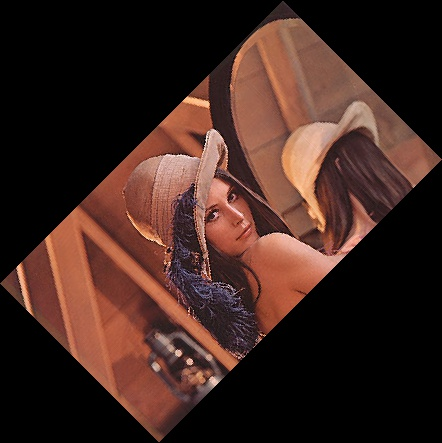## Coordinates of the center of rotation: `center`

You can specify the rotation center position with the parameter `center`. By default, `center` is the center of the image.

``````im_rotate = im.rotate(45, center=(0, 60))
``````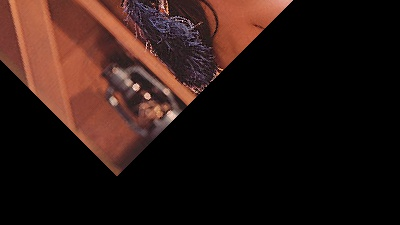In the case of `expand=True`, the output image area is determined assuming that the image is rotated about the image center.

``````im_rotate = im.rotate(45, center=(0, 60), expand=True)
``````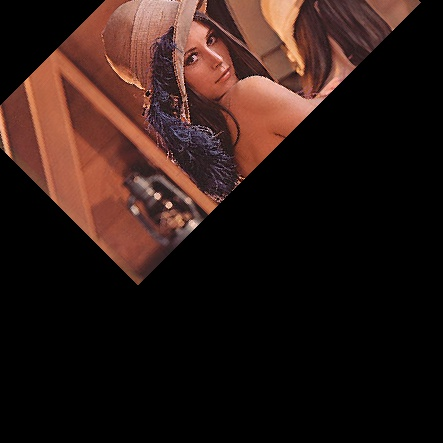## Translation: `translate`

You can translate before rotation with the parameter `translate`. `translate` is specified by `(translation distance in x direction, translation distance in y direction)`.

Without rotation:

``````im_rotate = im.rotate(0, translate=(100, 50))
``````Rotate 45 degrees and translate:

``````im_rotate = im.rotate(45, translate=(100, 50))
``````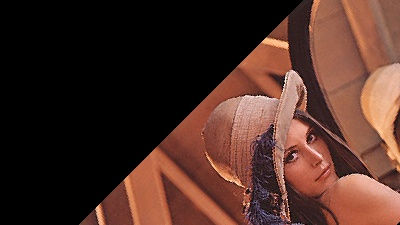If `expand=True`, the output image area is determined assuming that the image is rotated without translation.

``````im_rotate = im.rotate(45, translate=(100, 50), expand=True)
``````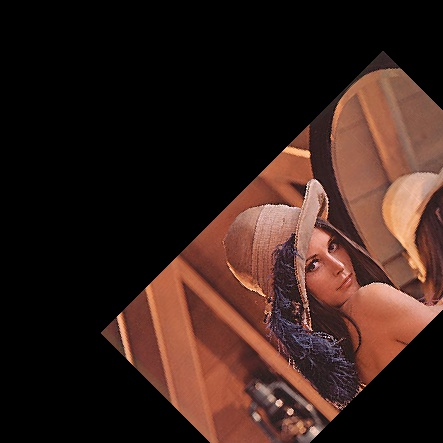## Outer Color: `fillcolor`

You can specify the outer color with the `fillcolor`. The default color is black.

In the case of RGB images, it is specified as a tuple of `(R, G, B)`.

``````im_rotate = im.rotate(45, fillcolor=(255, 128, 0), expand=True)
``````